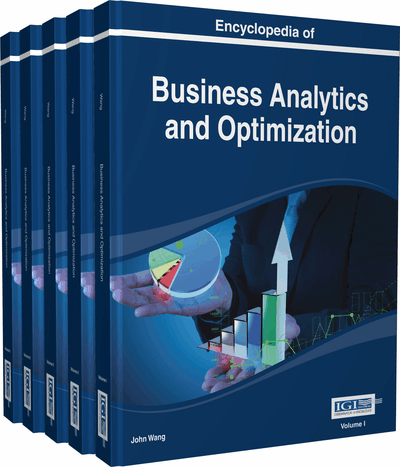# Constrained Nonlinear Optimization in Business

William P. Fox (Naval Postgraduate School, USA)
DOI: 10.4018/978-1-4666-5202-6.ch047

## Abstract

We present both classical analytical, numerical, and heuristic techniques to solve constrained optimization problems relating to business, industry, and government. We briefly discuss other methods such as genetic algorithm. Today's business environment has many resource challenges to their attempts to maximize profits or minimize costs for which constrained optimization might be used. Facility location and transportation networks techniques are often used as well as the traveling salesman problem.
Chapter Preview
Top

## Introduction

A company manufactures new smart-phones that are supposed to capture the market by storm. The two main inputs components of the new smart-phone are the circuit board and the relay switches that make the phone faster and smarter and give it more memory.

The number of smart-phones to be produced is estimated to equal to, where E is the number of smart-phones produced and a & b are the number of circuit broad hours and the number of relay hours worked, respectively. Such a function is known to economists as a Cobb–Douglas function. Laborers are paid by the type of work they do: the circuit boards and the relays for \$5 and \$10 an hour, respectively. We want to maximize the number of smart-phones to be made if we have \$150,000 to spend on these components in the short run.

Lagrange multipliers can be used to solve nonlinear optimization problems (NLPs) in which all the constraints are equality constrained. We consider the following type of NLPs as shown by Equation (1):

• Maximize (Minimize)z = f(x1, x2, x3, . . ., xn) (1)

Subject to

g1(x1, x2, . . ., xn) = b1g2(x1, x2, . . ., xn) = b2gm(x1, x2, . . ., xn) = bm

In our smart-phones example, we find we can build an equality constrained model. Our model is

Subject to:

5a + 10b = 150,000

Problems in business, industry, and government such as this can be modeled using constrained optimization. Our discussion includes equality constrained optimization, inequality constrained optimization, and some numerical methods to approximate solutions.

Top

## Background

The general constrained nonlinear programming (NLP) problem is to find x* so as to optimize f(x), x=(x1,x2,…,xn) subject to the constraints of the problem.

• Maximize or Minimizef(x1,x2,…,xn)

Subject tofor i = 1,2,…,m.

Classical constrained optimization appeared with equality constrains and Lagrange multiplier named for Joseph Lagrange in the late 1700’s. It was almost two hundred years later when Kuhn-Tucker (1951) presented their famous Kuhn-Tucker (KT) conditions. Scholars later found that Karsuh (1939) had done considerable work on his thesis in the area of constrained optimization and thus, was added his name was added to create the Karsh-Kuhn-Tucker (KKT) conditions. Bellman (1952; 1957) created dynamic programming in the 1950’s to handle sequential constraints in optimization.

## Key Terms in this Chapter

Decision Variables: the variables that change that affect the solution.

Constraints: A resource constraint is one that either limits the amount of a resource or sets a minimum to amount of a resource requirement.

Lagrange Multiplier: The Lagrange multiplier, ?, is used in sensitivity analysis in Optimization problems. In general the objective function changes by the value of ? for each unit change in the resource.

Initial Values: Staring values for numerical methods. Default values are usually all 0’s or all 1’s but care must be taken to insure convergence.

Lagrange: A nonlinear optimization methodology that involved equality constraints.

Binding Constraint: A constraint that is satisfied at equality.

Numerical Methods: When a function cannot be solved in closed form an appropriate numerical technique may be used to approximate the solution.

Kuhn-Tucker Conditions: A nonlinear optimization methodology that involved Inequality constraints.

Optimization: The process of maximizing or minimizing an objective function.

Feasible Region: The convex region bounded by the constraints.

## Complete Chapter List

Search this Book:
Reset